Courses

# Phase Modulation Electronics and Communication Engineering (ECE) Notes | EduRev

## Communication Theory

Created by: Machine Experts

## Electronics and Communication Engineering (ECE) : Phase Modulation Electronics and Communication Engineering (ECE) Notes | EduRev

The document Phase Modulation Electronics and Communication Engineering (ECE) Notes | EduRev is a part of the Electronics and Communication Engineering (ECE) Course Communication Theory.
All you need of Electronics and Communication Engineering (ECE) at this link: Electronics and Communication Engineering (ECE)

PHASE MODULATION:

Phase modulation (PM) is a form of modulation that represents information as variations in the instantaneous phase of a carrier wave.

Unlike its more popular counterpart, frequency modulation (FM), PM is not very widely used for radio transmissions. This is because it tends to require more complex receiving hardware and there can be ambiguity problems in determining whether, for example, the signal has changed phase by +180° or -180°. PM is used, however, in digital music synthesizers such as the Yamaha DX7, even though these instruments are usually referred to as "FM" synthesizers (both modulation types sound very similar, but PM is usually easier to implement in this area).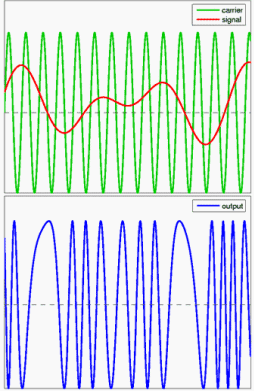An example of phase modulation. The top diagram shows the modulating signal superimposed on the carrier wave. The bottom diagram shows the resulting phase modulated signalPM changes the phase angle of the complex envelope in direct proportion to the message signal.

Suppose that the signal to be sent (called the modulating or message signal) is m(t) and the carrier onto which the signal is to be modulated is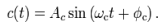Annotated:

carrier(time) = (carrier amplitude)*sin(carrier frequency*time + phase shift)

This makes the modulated signal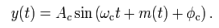This shows how m(t) modulates the phase - the greater m(t) is at a point in time, the greater the phase shift of the modulated signal at that point. It can also be viewed as a change of the frequency of the carrier signal, and phase modulation can thus be considered a special case of FM in which the carrier frequency modulation is given by the time derivative of the phase modulation.

The spectral behavior of phase modulation is difficult to derive, but the mathematics reveals that there are two regions of particular interest:

• For small amplitude signals, PM is similar to amplitude modulation (AM) and exhibits its unfortunate doubling of baseband bandwidth and poor efficiency.
• For a single large sinusoidal signal, PM is similar to FM, and its bandwidth is approximately ,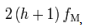where f= ωm / 2π and h is the modulation index defined below. This is also known as Carson's Rule for PM.

MODULATION INDEX:

As with other modulation indices, this quantity indicates by how much the modulated variable varies around its unmodulated level. It relates to the variations in the phase of the carrier signal:

h = Δ θ,

where Δθ is the peak phase deviation. Compare to the modulation index for frequency modulation.

Variable-capacitance diode phase modulator:

This circuit varies the phase between two square waves through at least 180°. This capability finds application in fixed-frequency, phase shift, resonant-mode converters. ICs such as the UC3875 usually only work up to about 500 kHz, whereas this circuit can be extended up to tens of megahertz. In addition, the circuit shown uses low-cost components. This example was used for a high-efficiency 2-MHz RF power supply.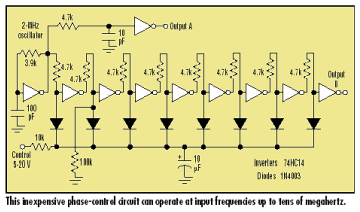The signal is delayed at each gate by the RC network formed by the 4.7k input resistor and capacitance of the 1N4003 diode. The capacitance of the diode, and hence delay, can be varied by controlling the reverse dc bias applied across the diode. The 100k resistor to ground at the input to the second stage corrects a slight loss of 1:1 symmetry. The fixed delay for output A adjusts the phase to be approximately in phase at a 5-V bias.

Note that the control voltage should not drop below approximately 3 V, because the diodes will start to be forward-biased and the signal will be lost.

Offer running on EduRev: Apply code STAYHOME200 to get INR 200 off on our premium plan EduRev Infinity!

,

,

,

,

,

,

,

,

,

,

,

,

,

,

,

,

,

,

,

,

,

;